• python时间序列分析
2022-04-16 22:20:05

## 时间序列分析 | Python实现时间序列数据分析

### 基本介绍

时间序列的处理是传统经济学里面的一个重要篇章，在数据科学和机器学习的背景下，时间序列分析所包含的内容更加复杂。

• 计量经济学里的时间序列特指一元时间序列，也就是数据包含两列，第一列是时间戳，第二列是观察对象。这属于比较经典的时间序列。有时候你会注意到一些时间序列的模型或者算法，比如ARIMA，prophet等，都是针对这类时间序列。
• 商业里面的交易历史信息也是一元时间序列。工业领域中，一些监测数据，比如天气温度，也是一元时间序列。但是时间序列不止有一元时间序列，当同一个时间戳对应的观测对象不只一个时，我们就有了多元时间序列。比如某个城市的空气PM2.5的预测，我们可以通过PM2.5的历史时间观测值来预测。我们也可以通过当天（或者近期）的其他观测对象来预测，比如风速，温度，湿度等。
• 多元时间序列在表现形式上就是数据包含多列（大于两列），第一列是时间戳，后面的列都是观察对象。当时间序列是多元时，很多经典的机器学习模型可以施展拳脚，比如回归模型&#x时序模型
更多相关内容
• 【项目实战】基于Python实现时间序列分析建模(ARIMA模型)项目实战 内容包括： 资料说明：包括数据集+源代码+PDF文档说明+代码视频讲解。 资料内容包括： 1)项目背景； 2)数据收集； 3)数预处理； 4)RBF神经网络...
• Datawhale干货译者：陈超，北京大学，数据派THU 本文约7500字，建议阅读20+分钟本文介绍了时间序列的定义、特征并结合实例给出了时间序列Python中评价指标和方法。...

Datawhale干货

译者：陈超北京大学，数据派THU



本文约7500字，建议阅读20+分钟本文介绍了时间序列的定义、特征并结合实例给出了时间序列在Python中评价指标和方法。


时间序列是在规律性时间间隔上记录的观测值序列。本指南将带你了解在Python中分析给定时间序列的特征的全过程。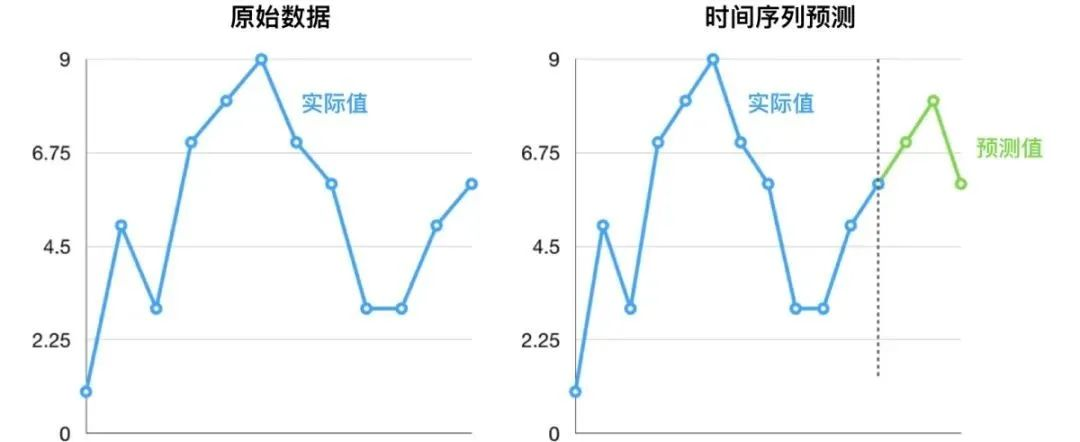主要内容

1. 什么是时间序列？

2. 如何在Python中导入时间序列？

3. 什么是面板数据？

4. 时间序列可视化

5. 时间序列的模式

6. 时间序列的加法和乘法

7. 如何将时间序列分解？

8. 平稳和非平稳时间序列

9. 如何获取平稳的时间序列？

10. 如何检验平稳性？

11. 白噪音和平稳序列的差异是什么？

12. 如何去除时间序列的线性分量？

13. 如何消除时间序列的季节性？

14. 如何检验时间序列的季节性？

15. 如何处理时间序列中的缺失值？

16. 什么是自回归和偏自回归函数？

17. 如何计算偏自回归函数？

18. 滞后图

19. 如何估计时间序列的预测能力？

20. 为什么以及怎样使时间序列平滑？

21. 如何使用Granger因果检验来获知时间序列是否对预测另一个序列帮助？

22. 下一步是什么?

1. 什么是时间序列?

时间序列是在规律性时间间隔记录的观测值序列。

依赖于观测值的频率，典型的时间序列可分为每小时、每天、每周、每月、每季度和每年为单位记录。有时，你可能也会用到以秒或者分钟为单位的时间序列，比如，每分钟用户点击量和访问量等等。

1.1 为什么要分析时间序列呢？

因为它是你做序列预测前的一步准备过程。而且，时间序列预测拥有巨大的商业重要性，因为对商业来说非常重要的需求和销量、网站访问人数、股价等都是时间序列数据。

1.2 所以时间序列分析包括什么内容呢？

时间序列分析包括理解序列内在本质的多个方面以便于你可更好地了解如何做出有意义并且精确的预测。

## 2. 如何在Python中导入时间序列？

所以怎样导入时间序列数据呢？典型的时间序列数据以.csv格式或者其他表格形式存储，包括两列：日期和测量值。

from dateutil.parser import parse
import matplotlib as mpl
import matplotlib.pyplot as plt
import seaborn as sns
import numpy as np
import pandas as pd
plt.rcParams.update({'figure.figsize': (10, 7), 'figure.dpi': 120})

# Import as Dataframe
df = pd.read_csv('https://raw.githubusercontent.com/selva86/datasets/master/a10.csv', parse_dates=['date'])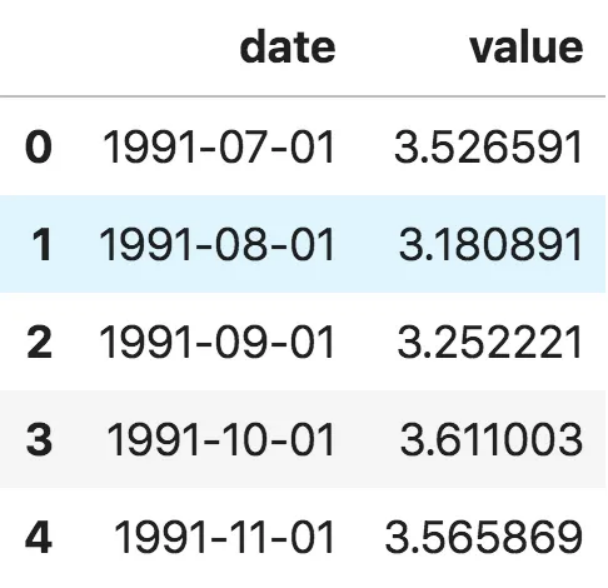数据框时间序列

ser = pd.read_csv('https://raw.githubusercontent.com/selva86/datasets/master/a10.csv', parse_dates=['date'], index_col='date')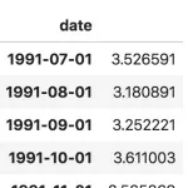时间序列

注意，在此序列当中，‘value’列的位置高于date以表明它是一个序列。

3. 什么是面板数据？

面板数据也是基于时间的数据集。

差异在于，除了时间序列，它也包括同时测量的一个或多个相关变量。

通常来看，面板数据当中的列包括了有助于预测Y的解释型变量，假设这些列将在未来预测阶段有用。

面板数据的例子如下：

# dataset source: https://github.com/rouseguy
df = df.loc[df.market=='MUMBAI', :]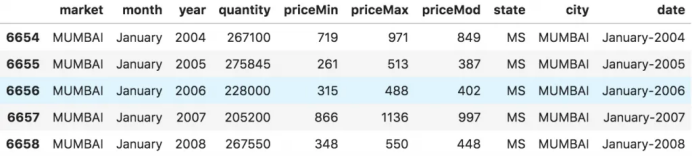面板数据

## 4. 时间序列可视化

让我们用matplotlib来对序列进行可视化。

# Time series data source: fpp pacakge in R.
import matplotlib.pyplot as plt
df = pd.read_csv('https://raw.githubusercontent.com/selva86/datasets/master/a10.csv', parse_dates=['date'], index_col='date')

# Draw Plot
def plot_df(df, x, y, title="", xlabel='Date', ylabel='Value', dpi=100):
plt.figure(figsize=(16,5), dpi=dpi)
plt.plot(x, y, color='tab:red')
plt.gca().set(title=title, xlabel=xlabel, ylabel=ylabel)
plt.show()

plot_df(df, x=df.index, y=df.value, title='Monthly anti-diabetic drug sales in  Australia from 1992 to 2008.')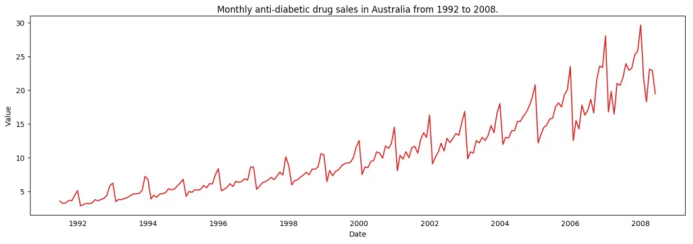时间序列可视化

因为所有的值都是正值，你可以在Y轴的两侧进行显示此值以强调增长。

# Import data
df = pd.read_csv('datasets/AirPassengers.csv', parse_dates=['date'])
x = df['date'].values
y1 = df['value'].values

# Plot
fig, ax = plt.subplots(1, 1, figsize=(16,5), dpi= 120)
plt.fill_between(x, y1=y1, y2=-y1, alpha=0.5, linewidth=2, color='seagreen')
plt.ylim(-800, 800)
plt.title('Air Passengers (Two Side View)', fontsize=16)
plt.hlines(y=0, xmin=np.min(df.date), xmax=np.max(df.date), linewidth=.5)
plt.show()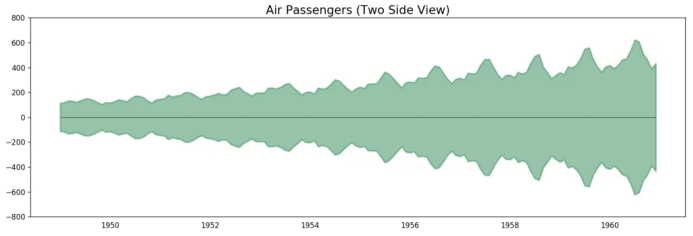航空乘客数据——两侧序列

因为这是一个月度时间序列，每年遵循特定的重复模式，你可以把每年作为一个单独的线画在同一张图上。这可以让你同时比较不同年份的模式。

4.1 时间序列的季节图

# Import Data
df = pd.read_csv('https://raw.githubusercontent.com/selva86/datasets/master/a10.csv', parse_dates=['date'], index_col='date')
df.reset_index(inplace=True)

# Prepare data
df['year'] = [d.year for d in df.date]
df['month'] = [d.strftime('%b') for d in df.date]
years = df['year'].unique()

# Prep Colors
np.random.seed(100)
mycolors = np.random.choice(list(mpl.colors.XKCD_COLORS.keys()), len(years), replace=False)

# Draw Plot
plt.figure(figsize=(16,12), dpi= 80)
for i, y in enumerate(years):
if i > 0:
plt.plot('month', 'value', data=df.loc[df.year==y, :], color=mycolors[i], label=y)
plt.text(df.loc[df.year==y, :].shape-.9, df.loc[df.year==y, 'value'][-1:].values, y, fontsize=12, color=mycolors[i])

# Decoration
plt.gca().set(xlim=(-0.3, 11), ylim=(2, 30), ylabel='$Drug Sales$', xlabel='$Month$')
plt.yticks(fontsize=12, alpha=.7)
plt.title("Seasonal Plot of Drug Sales Time Series", fontsize=20)
plt.show()药品销售的季节图

每年二月会迎来药品销售的急速下降，而在三月会再度上升，接下来的4月又开始下降，以此类推。很明显，该模式在特定的某一年中重复，且年年如此。

然而，随着年份推移，药品销售整体增加。你可以很好地看到该趋势并且在年份箱线图当中看到它是怎样变化的。同样地，你也可以做一个月份箱线图来可视化月度分布情况。

4.2 月度（季节性）箱线图和年度（趋势）分布

你可以季节间隔将数据分组，并看看在给定的年份或月份当中值是如何分布的，以及随时间推移它们是如何比较的。

# Import Data
df = pd.read_csv('https://raw.githubusercontent.com/selva86/datasets/master/a10.csv', parse_dates=['date'], index_col='date')
df.reset_index(inplace=True)

# Prepare data
df['year'] = [d.year for d in df.date]
df['month'] = [d.strftime('%b') for d in df.date]
years = df['year'].unique()

# Draw Plot
fig, axes = plt.subplots(1, 2, figsize=(20,7), dpi= 80)
sns.boxplot(x='year', y='value', data=df, ax=axes)
sns.boxplot(x='month', y='value', data=df.loc[~df.year.isin([1991, 2008]), :])

# Set Title
axes.set_title('Year-wise Box Plot\n(The Trend)', fontsize=18);
axes.set_title('Month-wise Box Plot\n(The Seasonality)', fontsize=18)
plt.show()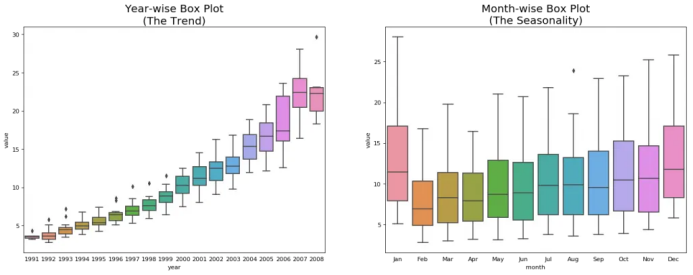年度和月度箱线图

箱线图将年度和月度分布变得很清晰。并且，在阅读箱线图当中，12月和1月明显有更高的药品销售量，可被归因于假期折扣季。

到目前为止，我们已经看到了识别模式的相似之处。现在怎样才能从通常模式当中找到离群值呢？

## 5. 时间序列的模式

任何时间序列都可以被分解为如下的部分：基线水平+趋势+季节性+误差

当在时间序列当中观测到增加或降低的斜率时，即可观测到相应的趋势。然而季节性只有在由于季节性因素导致不同的重复模式在规律性的间隔之间被观测到时才能发现。可能是由于当年的特定月份，特定月份的某一天、工作日或者甚至是当天某个时间。

然而，并不是所有时间序列必须有一个趋势和/或季节性。时间序列可能没有不同的趋势但是有一个季节性。反之亦然。

所以时间序列可以被看做是趋势、季节性和误差项的整合。

fig, axes = plt.subplots(1,3, figsize=(20,4), dpi=100)
pd.read_csv('https://raw.githubusercontent.com/selva86/datasets/master/guinearice.csv', parse_dates=['date'], index_col='date').plot(title='Trend Only', legend=False, ax=axes)

pd.read_csv('https://raw.githubusercontent.com/selva86/datasets/master/sunspotarea.csv', parse_dates=['date'], index_col='date').plot(title='Seasonality Only', legend=False, ax=axes)

pd.read_csv('https://raw.githubusercontent.com/selva86/datasets/master/AirPassengers.csv', parse_dates=['date'], index_col='date').plot(title='Trend and Seasonality', legend=False, ax=axes)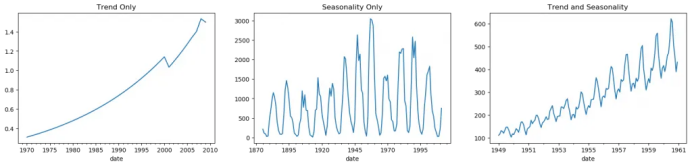时间序列中的模式

另一个需要考虑的方面是循环的行为。当序列当中上升和下降模式并不在固定的日历间隔出现时，就会出现循环的行为。需注意不要混淆循环的效应和季节的效应。

所以，怎样区分循环的和季节性的模式呢？

如果模式不是基于固定的日历频率，那它就是循环的。因为，循环效应不像季节性那样受到商业和其他社会经济因素的影响。

## 6. 时间序列的加法和乘法

基于趋势和季节性的本质，时间序列以加法或乘法的形式建模，其中序列里的每个观测值可被表达为成分的和或者积：

加法时间序列：值=基线水平+趋势+季节性+误差

乘法时间序列：值=基线水平*趋势*季节性*误差

## 7. 怎样分解时间序列的成分？

你可以通过将序列作基线水平，趋势，季节性指数和残差的加法或乘法组合来实现一个经典的时间序列分解。

statsmodels包里的seasonal_decompose使用起来非常方便。

from statsmodels.tsa.seasonal import seasonal_decompose
from dateutil.parser import parse

# Import Data
df = pd.read_csv('https://raw.githubusercontent.com/selva86/datasets/master/a10.csv', parse_dates=['date'], index_col='date')

# Multiplicative Decomposition
result_mul = seasonal_decompose(df['value'], model='multiplicative', extrapolate_trend='freq')

# Plot
plt.rcParams.update({'figure.figsize': (10,10)})
result_mul.plot().suptitle('Multiplicative Decompose', fontsize=22)
plt.show()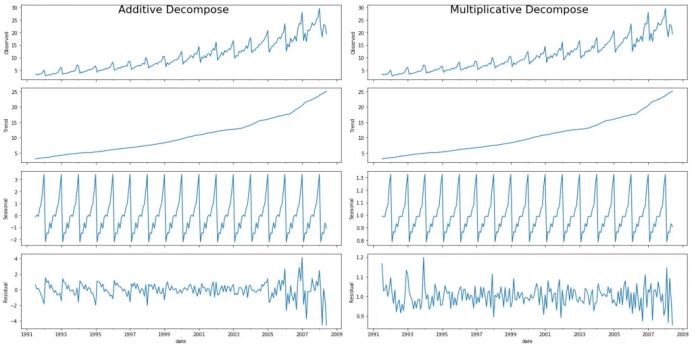加法和乘法分解

在序列开始时，设置extrapolate_trend='freq' 来注意趋势和残差中缺失的任何值。

如果你仔细看加法分解当中的残差，它有一些遗留模式。乘法分解看起来非常随意，这很好。所以理想状况下，乘法分解应该在这种特定的序列当中优先选择。

趋势，季节性和残差成分的数值输出被存储在result_mul 当中。让我们提取它们并导入数据框中。

# Extract the Components ----
# Actual Values = Product of (Seasonal * Trend * Resid)
df_reconstructed = pd.concat([result_mul.seasonal, result_mul.trend, result_mul.resid, result_mul.observed], axis=1)
df_reconstructed.columns = ['seas', 'trend', 'resid', 'actual_values']


如果你检查一下seas, trend 和 resid列的乘积，应该确实等于actual_values。

## 8. 平稳和非平稳时间序列

平稳性是时间序列的属性之一。平稳序列的值不是时间的函数。

也就是说，这种序列的统计属性例如均值，方差和自相关是随时间不变的常数。序列的自相关只是与前置值的相关，之后会详细介绍。

平稳时间序列也没有季节效应。

所以如何识别一个序列是否平稳呢？让我们通过实例来展示一下：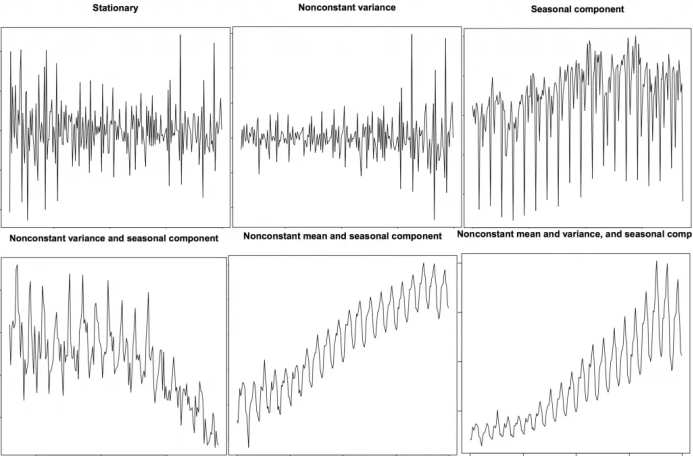平稳和非平稳时间序列

上图来自R语言的 TSTutorial。

所以为什么平稳序列是重要的呢？为什么我要提到它？

我将展开讲一下，但是要理解它只是有可能通过使用特定的转换方法实现任何时间序列的平稳化。大多数统计预测方法都用于平稳时间序列。预测的第一步通常是做一些转换将非平稳数据转化为平稳数据。

## 9. 如何获取平稳的时间序列？

你可以通过以下步骤实现序列的平稳化：

1. 差分序列（一次或多次）；

2. 对序列值进行log转换；

3. 对序列值去n次根式值；

4. 结合上述方法。

实现数据平稳化最常见也最方便的方法是对序列进行差分至少一次，直到它变得差不多平稳为止。

9.1 所以什么是差分？

如果Y_t是t时刻的Y值，那么第一次差分Y = Yt – Yt-1。在简化的格式当中，差分序列就是从当前值中减去下一个值。

如果第一次差分不能使数据平稳，你可以第二次差分，以此类推。

例如，考虑如下序列: [1, 5, 2, 12, 20]

一次差分: [5-1, 2-5, 12-2, 20-12] = [4, -3, 10, 8]

二次差分: [-3-4, -10-3, 8-10] = [-7, -13, -2]

## 9.2 为什么要在预测之前将非平稳数据平稳化？

预测平稳序列相对容易，预测也相对更可靠。

一个重要的原因是自回归预测模型必须是利用序列自身的滞后量作为预测变量的线性回归模型。

我们知道线性回归在预测变量（X变量）与其他变量不相关时效果最佳。所以序列平稳化也因为移除所有持续的自相关而解决了这个问题，因此使得模型中的预测变量（序列的滞后值）几乎独立。

现在我们已经建立了序列平稳化非常重要的概念，那怎样检验给定序列是否平稳化呢？

## 10. 怎样检验平稳性?

序列的平稳性可以通过之前我们提到的序列图看出来。

另外一种方法是将序列分成2或多个连续的部分，计算概要统计量例如均值，方差和自相关。如果统计量显著差异，序列可能不是平稳的。

尽管如此，你需要一个方法来从量化的角度判断一个给定序列是否平稳。可以通过‘Unit Root Tests单位根检验’来实现。这里有多种变式，但这些检验都是用来检测时间序列是否非平稳并且拥有一个单位根。

有多种单位根检验的具体应用：

2. 科维亚特夫斯基-菲利普斯-施密特-辛-KPSS检验（趋势平稳性）；

3. 菲利普斯 佩龙检验（PP Test）。

from statsmodels.tsa.stattools import adfuller, kpss
df = pd.read_csv('https://raw.githubusercontent.com/selva86/datasets/master/a10.csv', parse_dates=['date'])

result = adfuller(df.value.values, autolag='AIC')
print(f'p-value: {result}')
for key, value in result.items():
print('Critial Values:')
print(f'   {key}, {value}')

# KPSS Test
result = kpss(df.value.values, regression='c')
print('\nKPSS Statistic: %f' % result)
print('p-value: %f' % result)
for key, value in result.items():
print('Critial Values:')
print(f'   {key}, {value}')
p-value: 1.0
Critial Values:
1%, -3.465620397124192
Critial Values:
5%, -2.8770397560752436
Critial Values:
10%, -2.5750324547306476

KPSS Statistic: 1.313675
p-value: 0.010000
Critial Values:
10%, 0.347
Critial Values:
5%, 0.463
Critial Values:
2.5%, 0.574
Critial Values:
1%, 0.739


## 11. 白噪音和平稳序列的差异是什么？

如平稳序列，白噪音也不是时间的函数，它的均值和方差并不随时间变化。但是它与平稳序列的差异在于，白噪音完全随机，均值为0。

无论怎样，在白噪音当中是没有特定模式的。如果你将FM广播的声音信号作为时间序列，你在频道之间的频段听到的空白声就是白噪音。

从数学上来看，均值为0的完全随机的数字序列是白噪音。

randvals = np.random.randn(1000)
pd.Series(randvals).plot(title='Random White Noise', color='k')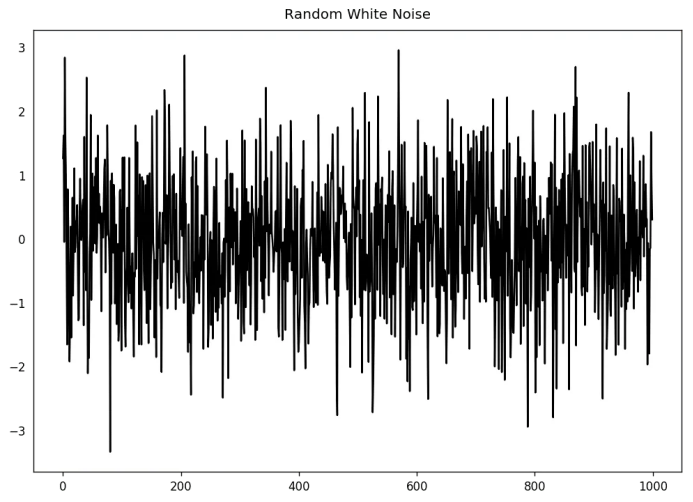随机白噪音

## 12. 怎样将时间序列去趋势化？

对时间序列去趋势就是从时间序列当中移除趋势成分。但是如何提取趋势呢？有以下几个方法。

1. 从时间序列当中减去最优拟合线。最佳拟合线可从以时间步长为预测变量获得的线性回归模型当中获得。对更复杂的模型，你可以使用模型中的二次项（x^2）；

2. 从我们之前提过的时间序列分解当中减掉趋势成分；

3. 减去均值；

4. 应用像Baxter-King过滤器(statsmodels.tsa.filters.bkfilter)或者Hodrick-Prescott 过滤器 (statsmodels.tsa.filters.hpfilter)来去除移动的平均趋势线或者循环成分。

让我们来用一下前两种方法。

# Using scipy: Subtract the line of best fit
from scipy import signal
df = pd.read_csv('https://raw.githubusercontent.com/selva86/datasets/master/a10.csv', parse_dates=['date'])
detrended = signal.detrend(df.value.values)
plt.plot(detrended)
plt.title('Drug Sales detrended by subtracting the least squares fit', fontsize=16)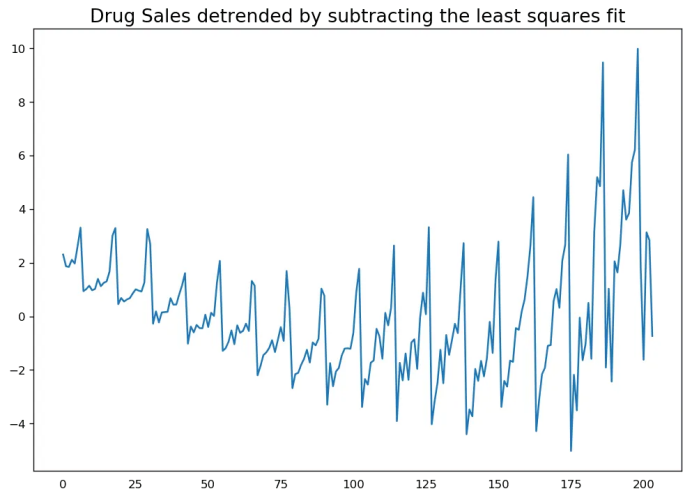通过减去最小二乘拟合来对时间序列去趋势化

# Using statmodels: Subtracting the Trend Component.
from statsmodels.tsa.seasonal import seasonal_decompose
df = pd.read_csv('https://raw.githubusercontent.com/selva86/datasets/master/a10.csv', parse_dates=['date'], index_col='date')
result_mul = seasonal_decompose(df['value'], model='multiplicative', extrapolate_trend='freq')
detrended = df.value.values - result_mul.trend
plt.plot(detrended)
plt.title('Drug Sales detrended by subtracting the trend component', fontsize=16)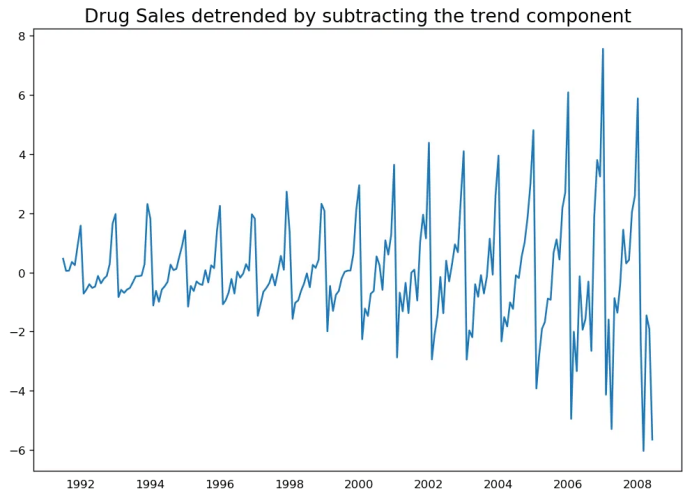通过减去趋势成分来去趋势化

## 13. 怎样对时间序列去季节化?

这里有多种方法对时间序列去季节化。以下就有几个：

1. 取一个以长度为季节窗口的移动平均线。这将在这个过程中使序列变得平滑；

2. 序列季节性差分（从当前值当中减去前一季节的值）；

3. 将序列值除以从STL分解当中获得的季节性指数。

如果除以季节性指数后仍没办法得到良好的结果，再试一下序列对数转换然后再做。你之后可以通过去指数恢复到原始尺度。

# Subtracting the Trend Component.
df = pd.read_csv('https://raw.githubusercontent.com/selva86/datasets/master/a10.csv', parse_dates=['date'], index_col='date')

# Time Series Decomposition
result_mul = seasonal_decompose(df['value'], model='multiplicative', extrapolate_trend='freq')

# Deseasonalize
deseasonalized = df.value.values / result_mul.seasonal

# Plot
plt.plot(deseasonalized)
plt.title('Drug Sales Deseasonalized', fontsize=16)
plt.plot()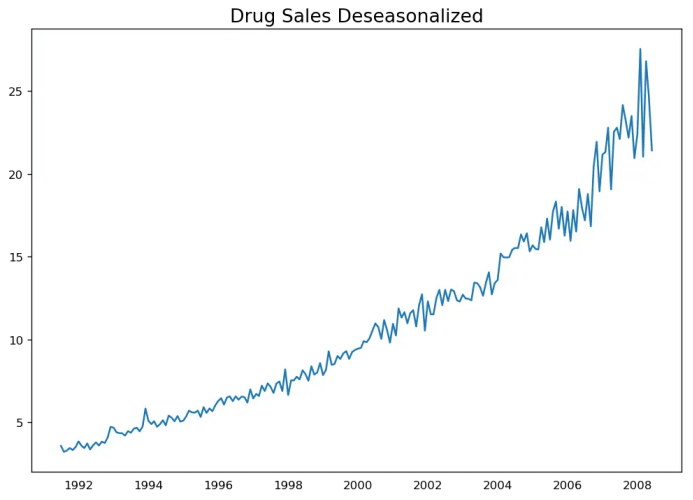时间序列去季节化

## 14. 怎样检验时间序列的季节性？

常见的方法是绘制序列并在固定的时间间隔内检查可重复的模式。所以，季节性的类型由钟表或日历决定：

1. 一天的每个小时；

2. 一月的每天；

3. 每周；

4. 每月；

5. 每年。

然而，如果你想要一个更权威的季节性检验，使用自回归函数（ACF）图。更多关于自回归的信息将在下一部分介绍。但是当强季节性模式出现时，ACF图通常揭示了在季节窗的倍数处明显的重复峰值。

例如，药品销售时间序列是每年都有重复模式的一个月度序列。所以，你可以看到第12,24和36条线等的峰值。

我必须警告你在现实世界的数据集当中，这样强的模式很难见到，并且有可能被各种噪音所扭曲，所以你需要一双仔细的眼睛来捕获这些模式。

from pandas.plotting import autocorrelation_plot

# Draw Plot
plt.rcParams.update({'figure.figsize':(9,5), 'figure.dpi':120})
autocorrelation_plot(df.value.tolist())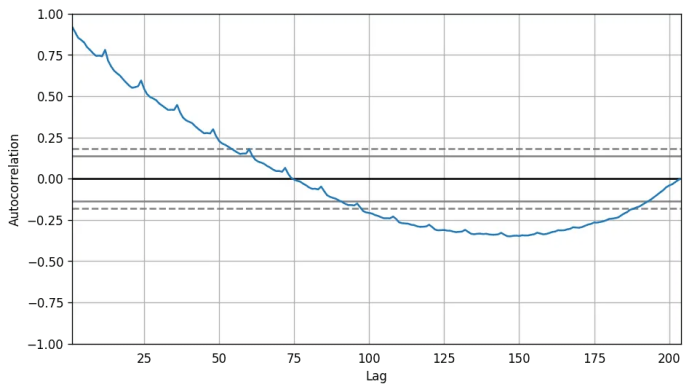自相关图

除此之外，如果你想做统计检验，CHT检验可以检验季节性差异是否对序列平稳化有必要。

## 15. 如何处理时间序列当中的缺失值？

有时，你的时间序列会有缺失日期/时间。那意味着，数据没有被捕获或者在那段时间内不可用。那些天的测量值有可能为0，你可以把那些时间段填充0。

其次，当处理时间序列时，你通常不应该用序列均值来替代缺失值，尤其是序列非平稳的时候，一个快捷粗略的处理方法来说你应该做的是向前填充之前的值。

然而，依赖于序列的本质，你想要在得出结论之前尝试多种方法。有效的缺失值处理方法有：

• 向后填充；

• 线性内插；

• 二次内插；

• 最邻近平均值；

• 对应季节的平均值。

为了衡量缺失值的表现，我在时间序列当中手动引入缺失值，使用上述方法处理并衡量处理值和真实值之间的均方误差。

# # Generate dataset
from scipy.interpolate import interp1d
from sklearn.metrics import mean_squared_error
df = pd.read_csv('datasets/a10_missings.csv', parse_dates=['date'], index_col='date')

fig, axes = plt.subplots(7, 1, sharex=True, figsize=(10, 12))
plt.rcParams.update({'xtick.bottom' : False})

## 1. Actual -------------------------------
df_orig.plot(title='Actual', ax=axes, label='Actual', color='red', style=".-")
df.plot(title='Actual', ax=axes, label='Actual', color='green', style=".-")
axes.legend(["Missing Data", "Available Data"])

## 2. Forward Fill --------------------------
df_ffill = df.ffill()
error = np.round(mean_squared_error(df_orig['value'], df_ffill['value']), 2)
df_ffill['value'].plot(title='Forward Fill (MSE: ' + str(error) +")", ax=axes, label='Forward Fill', style=".-")

## 3. Backward Fill -------------------------
df_bfill = df.bfill()
error = np.round(mean_squared_error(df_orig['value'], df_bfill['value']), 2)
df_bfill['value'].plot(title="Backward Fill (MSE: " + str(error) +")", ax=axes, label='Back Fill', color='firebrick', style=".-")

## 4. Linear Interpolation ------------------
df['rownum'] = np.arange(df.shape)
df_nona = df.dropna(subset = ['value'])
f = interp1d(df_nona['rownum'], df_nona['value'])
df['linear_fill'] = f(df['rownum'])
error = np.round(mean_squared_error(df_orig['value'], df['linear_fill']), 2)
df['linear_fill'].plot(title="Linear Fill (MSE: " + str(error) +")", ax=axes, label='Cubic Fill', color='brown', style=".-")

## 5. Cubic Interpolation --------------------
f2 = interp1d(df_nona['rownum'], df_nona['value'], kind='cubic')
df['cubic_fill'] = f2(df['rownum'])
error = np.round(mean_squared_error(df_orig['value'], df['cubic_fill']), 2)
df['cubic_fill'].plot(title="Cubic Fill (MSE: " + str(error) +")", ax=axes, label='Cubic Fill', color='red', style=".-")

# Interpolation References:
# https://docs.scipy.org/doc/scipy/reference/tutorial/interpolate.html
# https://docs.scipy.org/doc/scipy/reference/interpolate.html

## 6. Mean of 'n' Nearest Past Neighbors ------
def knn_mean(ts, n):
out = np.copy(ts)
for i, val in enumerate(ts):
if np.isnan(val):
n_by_2 = np.ceil(n/2)
lower = np.max([0, int(i-n_by_2)])
upper = np.min([len(ts)+1, int(i+n_by_2)])
ts_near = np.concatenate([ts[lower:i], ts[i:upper]])
out[i] = np.nanmean(ts_near)
return out

df['knn_mean'] = knn_mean(df.value.values, 8)
error = np.round(mean_squared_error(df_orig['value'], df['knn_mean']), 2)
df['knn_mean'].plot(title="KNN Mean (MSE: " + str(error) +")", ax=axes, label='KNN Mean', color='tomato', alpha=0.5, style=".-")

## 7. Seasonal Mean ----------------------------
def seasonal_mean(ts, n, lr=0.7):
"""
Compute the mean of corresponding seasonal periods
ts: 1D array-like of the time series
n: Seasonal window length of the time series
"""
out = np.copy(ts)
for i, val in enumerate(ts):
if np.isnan(val):
ts_seas = ts[i-1::-n]  # previous seasons only
if np.isnan(np.nanmean(ts_seas)):
ts_seas = np.concatenate([ts[i-1::-n], ts[i::n]])  # previous and forward
out[i] = np.nanmean(ts_seas) * lr
return out

df['seasonal_mean'] = seasonal_mean(df.value, n=12, lr=1.25)
error = np.round(mean_squared_error(df_orig['value'], df['seasonal_mean']), 2)
df['seasonal_mean'].plot(title="Seasonal Mean (MSE: " + str(error) +")", ax=axes, label='Seasonal Mean', color='blue', alpha=0.5, style=".-")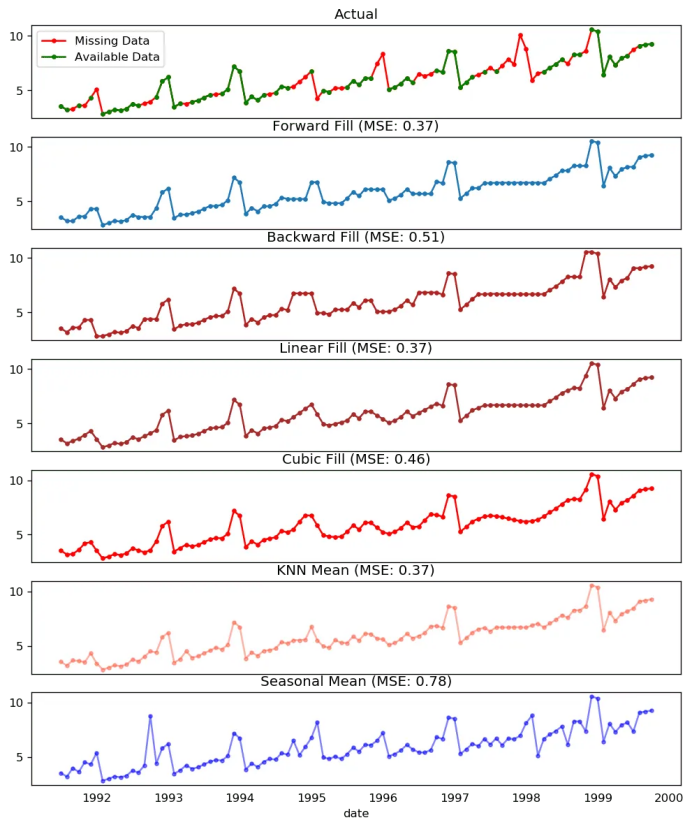缺失值处理

你也可以根据你想实现的精确程度考虑接下来的方法。

1. 如果你有解释变量，可以使用像随机森林或k-邻近算法的预测模型来预测它。

2. 如果你有足够多的过去观测值，可以预测缺失值。

3. 如果你有足够的未来观测值，回测缺失值。

4. 从之前的周期预测相对应的部分。

## 16. 什么是自相关和偏自相关函数？

自相关是序列和自己滞后量的简单相关。如果序列显著自相关，均值和序列之前的值（滞后量）可能对预测当前值有帮助。

偏自相关也会传递相似的信息但是它传递的是序列和它滞后量的纯粹相关，排除了其他中间滞后量对相关的贡献。

from statsmodels.tsa.stattools import acf, pacf
from statsmodels.graphics.tsaplots import plot_acf, plot_pacf

# Calculate ACF and PACF upto 50 lags
# acf_50 = acf(df.value, nlags=50)
# pacf_50 = pacf(df.value, nlags=50)

# Draw Plot
fig, axes = plt.subplots(1,2,figsize=(16,3), dpi= 100)
plot_acf(df.value.tolist(), lags=50, ax=axes)
plot_pacf(df.value.tolist(), lags=50, ax=axes)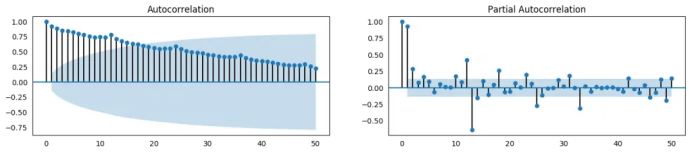自相关函数 和 偏自相关函数

## 17. 怎样计算偏自相关函数?

怎样计算偏自相关呢?

序列滞后量（k）的偏自相关是Y的自回归方程中滞后量的系数。Y的自回归方程就是Y及其滞后量作为预测项的线性回归。

For Example, if Y_t is the current series and Y_t-1 is the lag 1 of Y, then the partial autocorrelation of lag 3 (Y_t-3) is the coefficient $\alpha_3$ of Y_t-3 in the following equation:

例如，如果Y_t是当前的序列，Y_t-1是Y的滞后量1，那么滞后量3（Y_t-3）的偏自相关就是下面方程中Y_t-3的系数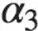: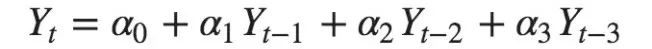自回归方程

## 18. 滞后图

滞后图是一个时间序列对其自身滞后量的散点图。它通常用于检查自相关。如果序列中存在如下所示的任何模式，则该序列是自相关的。如果没有这样的模式，这个序列很可能是随机的白噪声。

在下面太阳黑子面积时间序列的例子当中，随着n_lag增加，图越来越分散。

from pandas.plotting import lag_plot
plt.rcParams.update({'ytick.left' : False, 'axes.titlepad':10})

# Import

# Plot
fig, axes = plt.subplots(1, 4, figsize=(10,3), sharex=True, sharey=True, dpi=100)
for i, ax in enumerate(axes.flatten()[:4]):
lag_plot(ss.value, lag=i+1, ax=ax, c='firebrick')
ax.set_title('Lag ' + str(i+1))

fig.suptitle('Lag Plots of Sun Spots Area \n(Points get wide and scattered with increasing lag -> lesser correlation)\n', y=1.15)

fig, axes = plt.subplots(1, 4, figsize=(10,3), sharex=True, sharey=True, dpi=100)
for i, ax in enumerate(axes.flatten()[:4]):
lag_plot(a10.value, lag=i+1, ax=ax, c='firebrick')
ax.set_title('Lag ' + str(i+1))

fig.suptitle('Lag Plots of Drug Sales', y=1.05)
plt.show()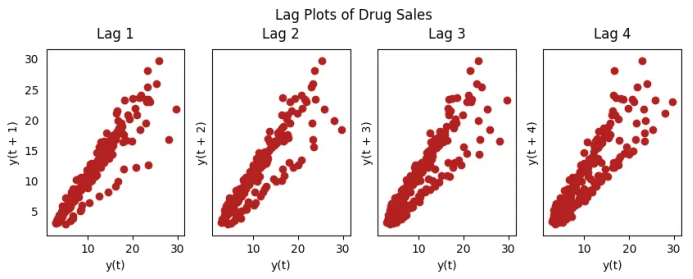药品销售的滞后图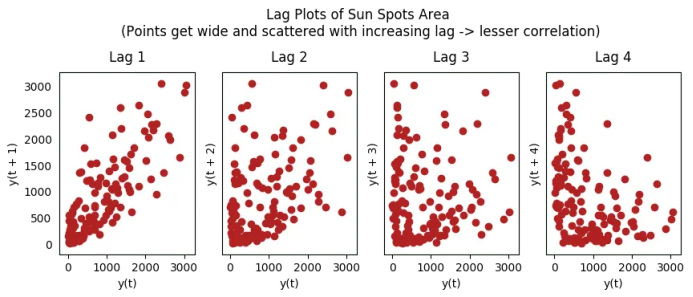太阳黑子的滞后图

## 19. 怎样估计时间序列的预测能力?

时间序列越有规律性和重复性的模式，越容易被预测。“近似熵”可用于量化时间序列波动的规律性和不可预测性。

近似熵越高，预测越难。另一个更好的选项是“样本熵”。

样本熵类似与近似熵，但是在估计小时间序列的复杂性上结果更一致。例如，较少样本点的随机时间序列 “近似熵”可能比一个更规律的时间序列更低，然而更长的时间序列可能会有一个更高的“近似熵”。

样本熵可以很好地处理这个问题。请看如下演示：

# https://en.wikipedia.org/wiki/Approximate_entropy
rand_small = np.random.randint(0, 100, size=36)
rand_big = np.random.randint(0, 100, size=136)

def ApEn(U, m, r):
"""Compute Aproximate entropy"""
def _maxdist(x_i, x_j):
return max([abs(ua - va) for ua, va in zip(x_i, x_j)])

def _phi(m):
x = [[U[j] for j in range(i, i + m - 1 + 1)] for i in range(N - m + 1)]
C = [len([1 for x_j in x if _maxdist(x_i, x_j) <= r]) / (N - m + 1.0) for x_i in x]
return (N - m + 1.0)**(-1) * sum(np.log(C))

N = len(U)
return abs(_phi(m+1) - _phi(m))

print(ApEn(ss.value, m=2, r=0.2*np.std(ss.value)))     # 0.651
print(ApEn(a10.value, m=2, r=0.2*np.std(a10.value)))   # 0.537
print(ApEn(rand_small, m=2, r=0.2*np.std(rand_small))) # 0.143
print(ApEn(rand_big, m=2, r=0.2*np.std(rand_big)))     # 0.716
0.6514704970333534
0.5374775224973489
0.0898376940798844
0.7369242960384561
# https://en.wikipedia.org/wiki/Sample_entropy
def SampEn(U, m, r):
"""Compute Sample entropy"""
def _maxdist(x_i, x_j):
return max([abs(ua - va) for ua, va in zip(x_i, x_j)])

def _phi(m):
x = [[U[j] for j in range(i, i + m - 1 + 1)] for i in range(N - m + 1)]
C = [len([1 for j in range(len(x)) if i != j and _maxdist(x[i], x[j]) <= r]) for i in range(len(x))]
return sum(C)

N = len(U)
return -np.log(_phi(m+1) / _phi(m))

print(SampEn(ss.value, m=2, r=0.2*np.std(ss.value)))      # 0.78
print(SampEn(a10.value, m=2, r=0.2*np.std(a10.value)))    # 0.41
print(SampEn(rand_small, m=2, r=0.2*np.std(rand_small)))  # 1.79
print(SampEn(rand_big, m=2, r=0.2*np.std(rand_big)))      # 2.42
0.7853311366380039
0.41887013457621214
inf
2.181224235989778

del sys.path


## 20. 为何要以及怎样对时间序列进行平滑处理?

时间序列平滑处理可能在以下场景有用：

• 在信号当中减小噪声的影响从而得到一个经过噪声滤波的序列近似。

• 平滑版的序列可用于解释原始序列本身的特征。

• 趋势更好地可视化。

怎样对序列平滑处理？让我们讨论一下以下方法：

1. 使用移动平均；

2. 做LOESS光滑（局部回归）；

3. 做LOWESS光滑（局部加权回归）。

移动均值就是定义宽度的滚动窗口的均值。但是你必须明智地选择窗口宽度，因为大范围窗口可能会造成序列过度平滑。例如，窗口大小等于季节持续时间时（例如：12为月度序列），将有效地抵消季节效应。

LOESS，局部回归的简写，适应于每个点邻近的多元回归。可通过statsmodels包使用，你可以使用frac参数确定被纳入拟合回归模型的邻近数据点的百分比来控制平滑度。

下载数据集: Elecequip.csv

from statsmodels.nonparametric.smoothers_lowess import lowess
plt.rcParams.update({'xtick.bottom' : False, 'axes.titlepad':5})

# Import
df_orig = pd.read_csv('datasets/elecequip.csv', parse_dates=['date'], index_col='date')

# 1. Moving Average
df_ma = df_orig.value.rolling(3, center=True, closed='both').mean()

# 2. Loess Smoothing (5% and 15%)
df_loess_5 = pd.DataFrame(lowess(df_orig.value, np.arange(len(df_orig.value)), frac=0.05)[:, 1], index=df_orig.index, columns=['value'])
df_loess_15 = pd.DataFrame(lowess(df_orig.value, np.arange(len(df_orig.value)), frac=0.15)[:, 1], index=df_orig.index, columns=['value'])

# Plot
fig, axes = plt.subplots(4,1, figsize=(7, 7), sharex=True, dpi=120)
df_orig['value'].plot(ax=axes, color='k', title='Original Series')
df_loess_5['value'].plot(ax=axes, title='Loess Smoothed 5%')
df_loess_15['value'].plot(ax=axes, title='Loess Smoothed 15%')
df_ma.plot(ax=axes, title='Moving Average (3)')
fig.suptitle('How to Smoothen a Time Series', y=0.95, fontsize=14)
plt.show()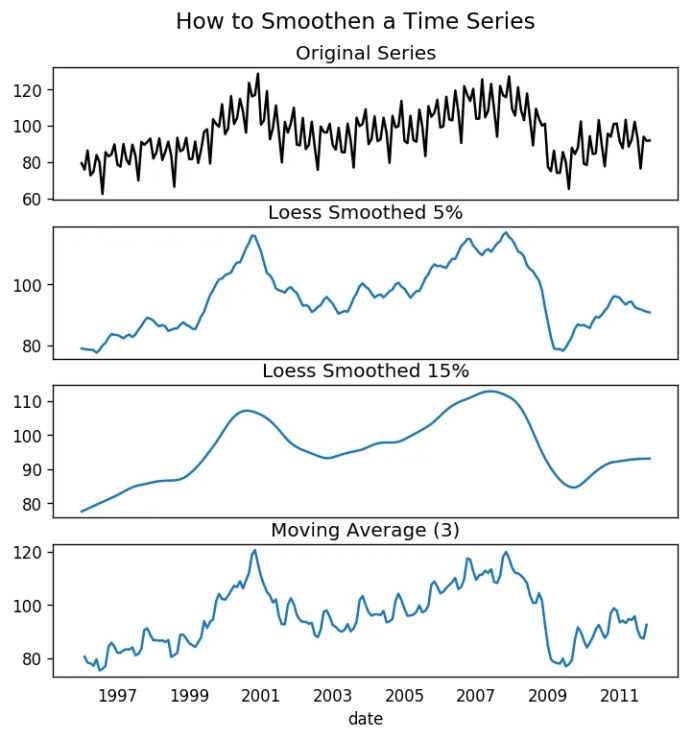平滑时间序列

## 21. 如何使用Granger因果检验得知是否一个时间序列有助于预测另一个序列？

Granger因果检验被用于检验是否一个时间序列可以预测另一个序列。Granger因果检验是如何工作的？

它基于如果X引起Y的变化，Y基于之前的Y值和之前的X值的预测效果要优于仅基于之前的Y值的预测效果。

所以需要了解Granger因果检验不能应用于Y的滞后量引起Y自身的变化的情况，而通常仅用于外源变量（不是Y的滞后量）。

它在statsmodel包中得到了很好的实现。它采纳2列数据的二维数组作为主要参数，被预测值是第一列，而预测变量（X）在第二列。

零假设检验：第二列的序列不能Granger预测第一列数据。如果p值小于显著性水平（0.05），你可以拒绝零假设并得出结论：X的滞后量确实有用。

第二个参数maxlag决定有多少Y的滞后量应该纳入检验当中。

from statsmodels.tsa.stattools import grangercausalitytests
df = pd.read_csv('https://raw.githubusercontent.com/selva86/datasets/master/a10.csv', parse_dates=['date'])
df['month'] = df.date.dt.month
grangercausalitytests(df[['value', 'month']], maxlag=2)
Granger Causality
number of lags (no zero) 1
ssr based F test:         F=54.7797 , p=0.0000  , df_denom=200, df_num=1
ssr based chi2 test:   chi2=55.6014 , p=0.0000  , df=1
likelihood ratio test: chi2=49.1426 , p=0.0000  , df=1
parameter F test:         F=54.7797 , p=0.0000  , df_denom=200, df_num=1

Granger Causality
number of lags (no zero) 2
ssr based F test:         F=162.6989, p=0.0000  , df_denom=197, df_num=2
ssr based chi2 test:   chi2=333.6567, p=0.0000  , df=2
likelihood ratio test: chi2=196.9956, p=0.0000  , df=2
parameter F test:         F=162.6989, p=0.0000  , df_denom=197, df_num=2


在上述例子当中，实际上所有检验的p值只能无限接近于0。所以“月份”实际上可以用于预测航空乘客的数量。

## 22. 下一步是什么？

这就是我们现在要说的。我们从非常基础的内容开始，理解了时间序列不同特征。一旦分析完成之后，接下来的一步是预测。

原文标题：

Time Series Analysis in Python – A Comprehensive Guide with Examples

原文链接：

https://www.machinelearningplus.com/time-series/time-series-analysis-python/

编辑：黄继彦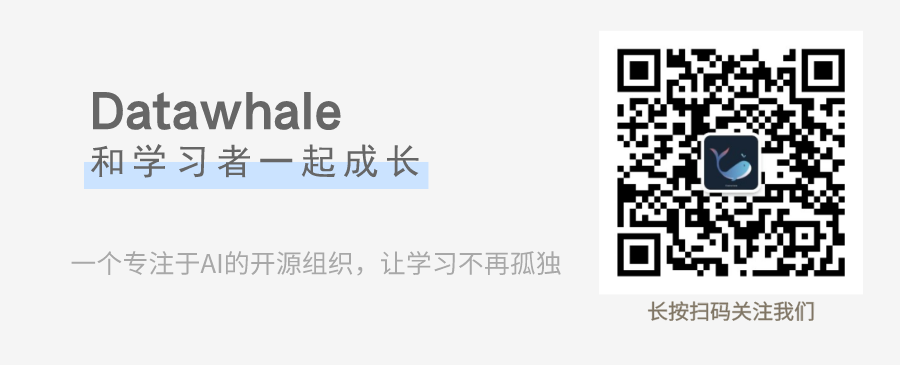干货学习，三连

展开全文python 机器学习
• 运用实际案例讲解如何使用Python编程的方式进行时间序列分析，包括： 自回归移动平均（ARMA）建模方法自回归差分移动平均（ARiMA）建模方法时间序列分解方法（趋势与季节性分析）授课内容简洁明了，突出重点，强调...python 大数据 时间序列分析
• Python时间序列分析 - 志光 - 博客园.html
• Python时间序列分析测试数据及Python时间序列分析代码
• 时间序列分析 | Python实现时间序列统计分析与建模 目录时间序列分析 | Python实现时间序列统计分析与建模基本介绍数据准备程序设计学习总结参考资料 基本介绍 本文介绍时间序列Python基本操作、统计分析、时间序列...

## 时间序列分析 | Python实现时间序列统计分析与建模

### 基本介绍

本文介绍时间序列Python基本操作、统计分析、时间序列分解与随机游走、统计建模分析。

### 数据准备

• Google Stocks Data：提供了长达十三年的股票数据。时间粒度精确到天，提供了每个股票每天的最高价，最低价格以及开盘价格等信息。
• Humidity in different world cities：提供了世界各地各个城市的大气湿度信息，时间粒度为分钟。

### 程序设计

# 读取数据

google 
展开全文python 统计分析
• dt1 %config InlineBackend.figure_format = 'retina' data.plot() plot_acf(data).show() print(u'原始序列的ADF检验结果为：', ADF(data[u'x'])) plot_pacf(data).show() 原始序列的ADF检验结果为： (-5....
import pandas as pd
import numpy as np
import matplotlib.pyplot as plt
import seaborn as sns
from statsmodels.graphics.tsaplots import plot_acf
from statsmodels.graphics.tsaplots import plot_pacf
from statsmodels.stats.diagnostic import acorr_ljungbox

%matplotlib inline

%pylab inline

#jupyter中文显示是方框，加入下面两行即可显示中文，若嫌麻烦，也可去网上搜索如何永久显示中文
plt.rcParams['font.sans-serif'] = ['SimHei'] #用来正常显示中文标签
plt.rcParams['axes.unicode_minus'] = False #用来正常显示负号
pylab.rcParams['figure.figsize'] = (10, 6)   #设置输出图片大小
sns.set(color_codes=True) #seaborn设置背景

np.random.seed(20211120)
x = np.random.normal(10, 5, 50)
t = np.linspace(1,20,50)

y = x + t

df  = {'x':list(x)}
data = pd.DataFrame(df)
dt1 = pd.date_range(start="1900", end="1950", freq="Y")
data.index = dt1

%config InlineBackend.figure_format = 'retina'
data.plot()

plot_acf(data).show()

plot_pacf(data).show()原始序列的ADF检验结果为： (-5.65584060487371, 9.62567971845179e-07, 0, 49, {‘1%’: -3.5714715250448363, ‘5%’: -2.922629480573571, ‘10%’: -2.5993358475635153}, 236.10635693304116)

展开全文python
• ## python时间序列分析

万次阅读 多人点赞 2018-03-05 22:45:49
本文转载自博客园大神“大熊猫淘沙”的一篇文章——python时间序列分析。 文章写的生动有趣干货满满，特此收藏转载一下。原文地址：https://www.cnblogs.com/foley/p/5582358.html 1. 什么是时间序列 1.1 环境...python datatime ARMA
• 在这里需要强调一点的是，时间序列分析并不是关于时间的回归，它主要是研究自身的变化规律的（这里不考虑含外生变量的时间序列）。 为什么用python 　用两个字总结“情怀”，爱屋及乌，个人比较喜欢python，就用...
• 时间序列分析 我的教授推荐了这本书。 我昨天读和《 。 两者都以自己的方式很好。 前者要先进得多，并提供一些您可能未曾接触过的想法，而后者则是中级的，但是有一些实际的例子，尽管这些例子有些简单化，而且...
• 意味着详尽无遗，如果您有任何遗漏，请随时编辑列表（将通过PR提出文件更改建议）机器学习，统计信息和分析库项目名称说明Arrow一种明智，人性化的创建，操作，格式化和转换日期的方法，时间和时间戳铯时间序列pla
• 介绍：金融市场大多数时间序列的波动往往表现为非周期性循环，重标极差分析法从标度不变性角度出发，不仅能有效计算非周期性循环的平均循环长度（避免传统谱分析弊端），而且还能分析周期性循环的固定周期。...时间序列分析
• 在该算法，train是时间序列示例的训练集，其中时间序列所属的类被附加到时间序列的末尾。test是相应的测试集，它所属于的类别就是我们想要预测的结果。在该算法，对于测试集中的每一个时间序列，每一遍搜索必须...
• 本文将介绍使用Python来完成时间序列分析ARIMA模型的完整步骤与流程 时间序列分析概念 **《时间序列分析》**是统计学的一个非常重要的分支，是以概率论与数理统计为基础、计算机应用为技术支撑，迅速发展起来的...python
• 3个Python时间序列分析工具包1. tsfresh2. tslearnsktime总结参考资料 时间序列分析是一种经典问题，常见的场景有时序预测、时序分裂、时序聚类、异常检测等。这里介绍三个时间序列分析相关的工具包： tsfresh ...时间序列分析 tsfresh
• 几个python库实现了离散小波变换。 但是，它们都不是，或者至少我不知道是针对科学用途的。 该库旨在填补这一空白，特别是考虑到Percival和Walden描述的离散小波变换。 该模块是从wmtsa Matlab工具箱...
• 何为时间序列分析： 时间序列经常通过折线图绘制。时间序列用于统计，信号处理，模式识别，计量经济学，数学金融，天气预报，地震预测，脑电图，控制工程，天文学，通信工程，以及主要涉及时间测量的任何应用科学和...python
• 常用的时间序列数据的分析两类：·趋势分解法简介：将时间序列分解为趋势、周期、随机三部分，并对前两个部分使用曲线进行拟合适合场景：适合所有类型的时间序列数据，需要事先判断走势及周期性·ARIMA法简介：根据...
• 购买课程后，添加小助手微信（微信号：csdn500）回复【唐宇迪】 进入学习群，获取唐宇迪老师答疑 Python时间序列分析视频培训教程，该课程以Python为核心工具，使用Pandas库进行时间序列的预处理与分析。详解时间...机器学习 机器学习实战 python机器学习
• Python时间序列分析与实战时间序列分析ARIMA自回归模型（AR）自回归模型的限制移动平均模型（MA）自回归移动平均模型（ARMA）ARIMA(p，d，q)模型自相关函数ACF(autocorrelation function)偏自相关函数(PACF)(partial...
• 在处理数据，遇到多年按月或按季节求均值的问题，在这里做一记录！ 1、加载nc数据 import xarray as xr ds=xr.open_dataset(r'E:\Allmode\salem_russia\russia_pr\salemtrans_0.5_pr_Amon_BCC-CSM2-MR_ssp126_r1i1...python
• 时间序列分析是数据科学家最常见的问题之一。 大多数时间序列解决方案涉及经济预测、资源需求预测、股票市场分析和销售分析。 如果从头开始，为大量与时间相关的数据开发复杂的模型对于程序员来说可能是一项艰巨的...人工智能 机器学习
• 时间序列分析 | Python时间序列预测理论一览 目录时间序列分析 | Python时间序列预测理论一览基本介绍特性描述建模方法规则介绍模型分类传统时序模型树模型线性回归神经网络模型学习总结 基本介绍 时间序列预测...时序模型 python
• 时间序列时间序列数据是一种非常重要的结构化数据形式，应用于：金融学、经济学、神经科学、物理学等多个领域。很多时间序列是固定频率的，数据点是根据某种规律定期出现的时间序列也可以是不定期的，没有固定的单位...
•python
• 显示这样的 'ARMA' object has no attribute 'resid' 请问uu们这个问题怎么解决呀 关于时间序列残差的问题python
• 因此，我获得了以传感器为基础的时间序列数据，该数据以秒为间隔进行测量，每个时间点的对应心率均采用Excel格式。我的目标是分析一段时间内是否存在任何趋势。当我将其导入Python时，可以看到一定数量，但看不到...
• 使用python 实现时间序列信号的频谱、倒频谱以及功率谱 ，资源以振动信号为例，并封装了相应的函数，可以见我的博客，马上就能理解...